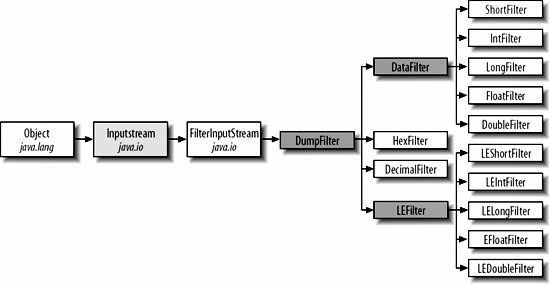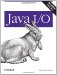# File Viewer, Part 3

In Chapter 4, I introduced a FileDumper program that could print the raw bytes of a file in ASCII, hexadecimal, or decimal. In this chapter, I'm going to expand that program so that it can interpret the file as containing binary numbers of varying widths. In particular, I'm going to make it possible to dump a file as shorts, unsigned shorts, ints, longs, floats, and doubles. Integer types may be either big-endian or little-endian. The main class, FileDumper3, is shown in Example 8-7. As in Chapter 4, this program reads a series of filenames and arguments from the command line in the main( ) method. Each filename is passed to a method that opens a file input stream from the file. Depending on the command-line arguments, a particular subclass of DumpFilter from Chapter 6 is selected and chained to the input stream. Finally, the StreamCopier.copy( ) method pours data from the input stream onto System.out.

Example 8-7. The FileDumper3 class

 ```import java.io.*; import com.elharo.io.*; public class FileDumper3 { public static final int ASC = 0; public static final int DEC = 1; public static final int HEX = 2; public static final int SHORT = 3; public static final int INT = 4; public static final int LONG = 5; public static final int FLOAT = 6; public static final int DOUBLE = 7; public static void main(String[] args) { if (args.length < 1) { System.err.println( "Usage: java FileDumper3 [-ahdsilfx] [-little] file1 file2..."); } boolean bigEndian = true; int firstFile = 0; int mode = ASC; // Process command-line switches. for (firstFile = 0; firstFile < args.length; firstFile++) { if (!args[firstFile].startsWith("-")) break; if (args[firstFile].equals("-h")) mode = HEX; else if (args[firstFile].equals("-d")) mode = DEC; else if (args[firstFile].equals("-s")) mode = SHORT; else if (args[firstFile].equals("-i")) mode = INT; else if (args[firstFile].equals("-l")) mode = LONG; else if (args[firstFile].equals("-f")) mode = FLOAT; else if (args[firstFile].equals("-x")) mode = DOUBLE; else if (args[firstFile].equals("-little")) bigEndian = false; } for (int i = firstFile; i < args.length; i++) { try { InputStream in = new FileInputStream(args[i]); dump(in, System.out, mode, bigEndian); if (i < args.length-1) { // more files to dump System.out.println( ); System.out.println("--------------------------------------"); System.out.println( ); } } catch (Exception ex) { System.err.println(ex); } } } public static void dump(InputStream in, OutputStream out, int mode, throws IOException { // The reference variable in may point to several different objects // within the space of the next few lines. We can attach // more filters here to do decompression, decryption, and more. if (bigEndian) { DataInputStream din = new DataInputStream(in); switch (mode) { case HEX: in = new HexFilter(in); break; case DEC: in = new DecimalFilter(in); break; case INT: in = new IntFilter(din); break; case SHORT: in = new ShortFilter(din); break; case LONG: in = new LongFilter(din); break; case DOUBLE: in = new DoubleFilter(din); break; case FLOAT: in = new FloatFilter(din); break; default: } } else { LittleEndianInputStream lin = new LittleEndianInputStream(in); switch (mode) { case HEX: in = new HexFilter(in); break; case DEC: in = new DecimalFilter(in); break; case INT: in = new LEIntFilter(lin); break; case SHORT: in = new LEShortFilter(lin); break; case LONG: in = new LELongFilter(lin); break; case DOUBLE: in = new LEDoubleFilter(lin); break; case FLOAT: in = new LEFloatFilter(lin); break; default: } } StreamCopier.copy(in, out); in.close( ); } } ```

The main( ) method of this class reads the command-line arguments and uses the switches to determine the format of the input data. The dump( ) method reads the mode and the endianness, selects the appropriate filter, and copies the input onto the output. Table 8-2 shows the command-line switches. Eight of these switches select a particular format. One of them, -little, specifies the endianness of the data. Since there's no difference between big-endian and little-endian ASCII, decimal, and hexadecimal dumps, a total of 12 different filters are used here. Two of the switches, the HexFilter and the DecimalFilter, were introduced in Chapter 6. They haven't changed.

Table 8-2. Command-line switches for FileDumper3

Switch

Format

-a

ASCII

-d

decimal dump

-h

-s

short

-i

int

-l

long

-f

float

-x

double

-little

little-endian

I've introduced ten new filters for big- and little-endian shorts, ints, longs, floats, and doubles. The big-endian filters read data from a data input stream. The little-endian filters read data from a little-endian input stream. To take advantage of code reuse, the big-endian filters are all subclasses of a new abstract subclass of DumpFilter called DataFilter, shown in Example 8-8. The little-endian filters are all subclasses of a new abstract subclass of DumpFilter called LEFilter, shown in Example 8-10. The hierarchy of these filters is shown in Figure 8-1.

Figure 8-1. Class hierarchy for filtersExample 8-8. DataFilter

 ```package com.elharo.io; import java.io.*; public abstract class DataFilter extends DumpFilter { // The use of DataInputStream here is a little forced. // It would be more natural (though more complicated) // to read the bytes and manually convert them to an int. private DataInputStream din; public DataFilter(DataInputStream din) { super(din); this.din = din; } public int available( ) throws IOException { return (buf.length - index) + in.available( ); } } ```

DataFilter makes sure that a data input stream is available to subclasses to read from. It also has enough information to provide a reasonable available( ) method. The actual implementation of the fill( ) method is left to specific subclasses like IntFilter. LEFilter, shwon in Example 8-9, is identical except for its use of a LittleEndianInputStream in place of a DataInputStream.

Example 8-9. LEFilter

 ```package com.elharo.io; import java.io.*; public abstract class LEFilter extends DumpFilter { private LittleEndianInputStream lin; public LEFilter(LittleEndianInputStream lin) { super(lin); this.lin = lin; } public int available( ) throws IOException { return (buf.length - index) + lin.available( ); } } ```

The concrete subclasses of these two classes are all very similar. Example 8-10 shows the simplest, IntFilter.

Example 8-10. IntFilter

 ```package com.elharo.io; import java.io.*; public class IntFilter extends DataFilter { public IntFilter(DataInputStream din) { super(din); } protected void fill( ) throws IOException { int number = din.readInt( ); String s = Integer.toString(number) + System.getProperty("line.separator", " "); byte[] b = s.getBytes("8859_1"); buf = new int[b.length]; for (int i = 0; i < b.length; i++) { buf[i] = b[i]; } } } ```

The fill( ) method reads an integer from the underlying DataInputStream din. That integer is converted to a string using the static Integer.toString( ) method. The string is then converted to bytes using the ISO 8859-1 (Latin-1) encoding.

The remaining DataFilter subclasses are very similar. For example, Example 8-11 shows the ShortFilter. Aside from the trivial difference in the class and constructor name, the only real difference is the use of readShort( ) instead of readInt() in the first line of the fill( ) method.

Example 8-11. ShortFilter

 ```package com.elharo.io; import java.io.*; public class ShortFilter extends DataFilter { public ShortFilter(DataInputStream din) { super(din); } protected void fill( ) throws IOException { int number = din.readShort( ); String s = Integer.toString(number) + System.getProperty("line.separator", " "); byte[] b = s.getBytes("8859_1"); buf = new int[b.length]; for (int i = 0; i < b.length; i++) { buf[i] = b[i]; } } } ```

The LongFilter, FloatFilter, and DoubleFilter are only slightly different, so I haven't put the source code in the book; it's available, with the rest of the examples, online. Likewise, I've omitted the similar set of filters for little-endian data. The little-endian filters all extend LEFilter; they are LEIntFilter, LEShortFilter, LELongFilter, LEFloatFilter, and LEDoubleFilter.

In later chapters, I'll add support for compressed and encrypted files, a graphical user interface, and various text interpretations of the data in a file. However, none of that will require changes to any of the filters we've developed here.Java I/O
ISBN: 0596527500
EAN: 2147483647
Year: 2004
Pages: 244